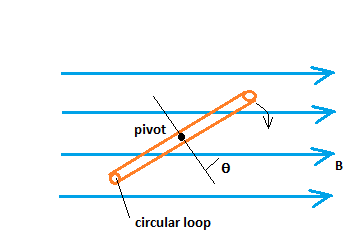# Figure is an edge on view of a 16 cm diameter circular lop rotating in a uniform B = 5.8 \times...

## Question:

Figure is an edge on view of a 16 cm diameter circular lop rotating in a uniform {eq}B = 5.8 \times 10^{-2} \space T {/eq} magnetic field. What is the magnetic flux through the loop when \theta is:

{eq}a. 0^\circ, \\ b. 30^\circ, \\ c. 60^\circ, \\ d. 90^\circ? {/eq}## Magnetic Flux

The number of magnetic field lines passing through a closed surface is known as magnetic flux. Mathematically Magnetic flux is given by

{eq}\begin{align} \Phi_B = B.A \cos \theta \end{align} {/eq}

Where B is the magnetic field in the region, A is the area of through which field lines pass, and {eq}\theta {/eq} is the angle between the magnetic field vector and the area vector.

Data Given

• Radius of the circular loop {eq}r = \frac{16 \ \rm cm}{2} = 8 \ \rm cm = 0.08 \ \rm m {/eq}
• Magnetic field in the region {eq}B = 5.8 \times 10^{-2} \ \rm T {/eq}

Part A) When {eq}\theta = 0^{\circ} {/eq} here the angle between the magnetic field and area vector will be {eq}90^{\circ} - \theta {/eq}

{eq}\begin{align} \Phi_B = B.A \cos (90^{\circ}- \theta) \\ \Phi_B = \pi \times (0.08 \ \rm m)^2 \times 5.8 \times 10^{-2} \ \rm T \times \cos (90^{\circ}-0^{\circ}) \\ \Phi_B = 0.0 \ \rm T.m^2 \end{align} {/eq}

Part B) When {eq}\theta = 30^{\circ} {/eq} here the angle between the magnetic field and area vector will be {eq}90^{\circ} - \theta {/eq}

{eq}\begin{align} \Phi_B = B.A \cos (90^{\circ}- \theta) \\ \Phi_B = \pi \times (0.08 \ \rm m)^2 \times 5.8 \times 10^{-2} \ \rm T \times \cos (90^{\circ}-30^{\circ}) \\ \Phi_B = 0.00058 \ \rm T.m^2 \end{align} {/eq}

Part C) When {eq}\theta = 60^{\circ} {/eq} here the angle between the magnetic field and area vector will be {eq}90^{\circ} - \theta {/eq}

{eq}\begin{align} \Phi_B = B.A \cos (90^{\circ}- \theta) \\ \Phi_B = \pi \times (0.08 \ \rm m)^2 \times 5.8 \times 10^{-2} \ \rm T \times \cos (90^{\circ}-60^{\circ}) \\ \Phi_B = 0.0010 \ \rm T.m^2 \end{align} {/eq}

Part D) When {eq}\theta = 90^{\circ} {/eq} here the angle between the magnetic field and area vector will be {eq}90^{\circ} - \theta {/eq}

{eq}\begin{align} \Phi_B = B.A \cos (90^{\circ}- \theta) \\ \Phi_B = \pi \times (0.08 \ \rm m)^2 \times 5.8 \times 10^{-2} \ \rm T \times \cos (90^{\circ}-90^{\circ}) \\ \Phi_B = 0.0012 \ \rm T.m^2 \end{align} {/eq}